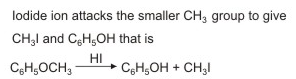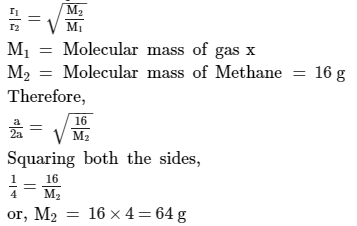Courses

# VITEEE Chemistry Test - 1

## 40 Questions MCQ Test VITEEE: Subject Wise and Full Length MOCK Tests | VITEEE Chemistry Test - 1

Description
This mock test of VITEEE Chemistry Test - 1 for JEE helps you for every JEE entrance exam. This contains 40 Multiple Choice Questions for JEE VITEEE Chemistry Test - 1 (mcq) to study with solutions a complete question bank. The solved questions answers in this VITEEE Chemistry Test - 1 quiz give you a good mix of easy questions and tough questions. JEE students definitely take this VITEEE Chemistry Test - 1 exercise for a better result in the exam. You can find other VITEEE Chemistry Test - 1 extra questions, long questions & short questions for JEE on EduRev as well by searching above.
QUESTION: 1

Solution:
QUESTION: 2

### Which of the following compounds will undergo self aldol condensation in the presence of cold dilute alkali?

Solution: For aldol condensation ,on carbonyl`s alpha position presence of sp3 hybridized carbon with alpha hydrogen must be there. In option a and c there is absence of sp3 hybridized alpha carbon. So answer is (b)
QUESTION: 3

### Which of the following reagent react differently with HCHO and CH₃CHO and CH₃COCH₃

Solution:
QUESTION: 4

The maximum amount of BaCl₄ precipitated on mixing BaCl₂ (0.5 M) with H₂SO₄ (1M) will correspond to

Solution:
QUESTION: 5

Wurtz reaction involves the interaction of alkyl halides in dry ether with

Solution:
QUESTION: 6

1-Butene may be converted to butane by reaction with

Solution:
QUESTION: 7

The product acetal is produced by reacting alcohol in the presence of dry HCl with

Solution:
QUESTION: 8

Silicon is

Solution:
QUESTION: 9

The protein which maintains blood sugar level is

Solution:
*Multiple options can be correct
QUESTION: 10

Alpha-Tocopherol is found in

Solution:

A nutrient that the body needs in small amounts to stay healthy and work the way it should. It is fat-soluble (can dissolve in fats and oils) and is found in seeds, nuts, leafy green vegetables, and vegetable oils. Alpha-tocopherol boosts the immune system and helps keep blood clots from forming.

Hence A and C

QUESTION: 11

Which is correct statement?

Solution:
QUESTION: 12

Methyl acetate will be obtained by reacting CH₃OH with

Solution:
QUESTION: 13

Dimerisation in carboxylic acid is due to

Solution:
QUESTION: 14

The compound which reacts with Fehling solution is

Solution:
QUESTION: 15

Which of the following gives blood red colour with KCNS ?

Solution:
QUESTION: 16

A cylinder filled with a movable piston contains liquid water in equilibrium with water vapour at 25oC. Which one of the following operations results in a decreace in the equilibrium vapour pressure ?

Solution:
QUESTION: 17

The internal energy of a subtance does not depend upon

Solution:
QUESTION: 18

The reaction is spontaneous if the cell potential is

Solution:
QUESTION: 19

Which one of the following is an example of exothermic reaction?

Solution:
QUESTION: 20
2-acetoxy benzoic acid is used as an
Solution:
QUESTION: 21

Which of the following is not aromatic ?

Solution:
QUESTION: 22

In the silver plating of copper, K[Ag(CN)₂] is used insead of AgNO₃. The reason is

Solution:
QUESTION: 23

Which of the following simple ions will not form complex ion with NH₃?

Solution: Pb2+ ion do not form complex with ammonia. It precipitate in form of a white salt rather than forming complex.Hence, correct answer is A.
QUESTION: 24

In the presence of acid, the hydrolysis of methyl cyanide gives

Solution:
QUESTION: 25

Which of the following compound gives dye test ?

Solution:
QUESTION: 26

When quantity of electricity passed is one faraday then the mass deposited at the electrode is equal to

Solution:
QUESTION: 27

Electrode potentials(Eored) of 4 elemnets A,B,C,D are -1.36V, - 0.32V, 0V, -1.26 V respectively. The decreasing reactivity order of these elements is

Solution: It is because among the positive potential higher positive will be more reactive . among negative least negative will be more reactive .
QUESTION: 28

Same amount of electric current is passed through solutions of AgNO₃ and HCI. If 1.08 g of silver is obtained in the first case, the volume of hydrogen liberated at S.T.P. in the second case is

Solution:
QUESTION: 29

Heating methyl phenyl ether with HI gives

Solution:QUESTION: 30
When the densities of the ore particles and gangue particles are different, then the ore is concentrated by
Solution:
QUESTION: 31

The rate of diffusion of methane at a given temperature is twice that of a gas X. The molecular weight of X is

Solution:
Let rate of diffusion of gas x, r1 = a
Therefore, rate of diffusion of methane,r2 = 2 a
According to Graham's Law of DiffusionQUESTION: 32

A pseudo solid is

Solution:
QUESTION: 33

The most stable hydride is

Solution: NH3 is the most stable hydride among the hydrides of group 15 elements because the strength of E-H (Here E = N, P, As, Sb, or Br) bond decreases down the group due to increase in size of the central atom.
QUESTION: 34
Phosphorus has the oxidation state of +3 in
Solution:
QUESTION: 35

What is hybridization of P in PCl₅?

Solution:
QUESTION: 36

The packing fraction for a body centred cubic is

Solution:
QUESTION: 37

Which of following pair is enantiomer?

Solution:
QUESTION: 38

Adsorption due to strong chemical forces is called

Solution:
QUESTION: 39

From a solution of CuSO₄, the metal used to recover copper is

Solution:
QUESTION: 40

A reagent that can separate Fe and Zn is

Solution: